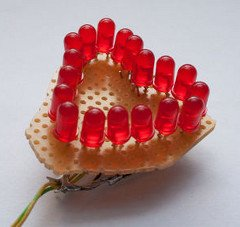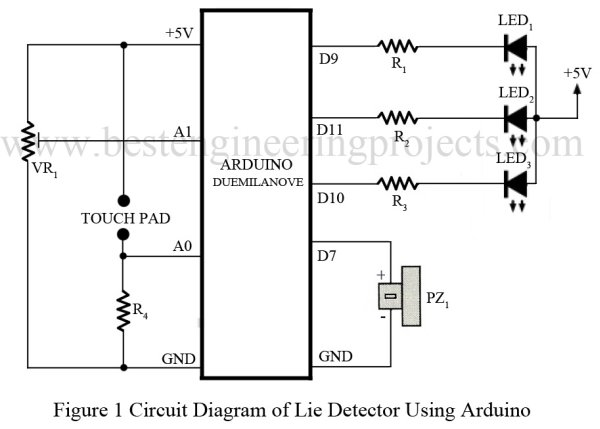# Arduino Based Lie Detector

We have previously posted the the project lie detector, now here is is also a lie detector using arduino. Here is a simple tutorial to build a simple lie detector which will give visual indication through LED arrangement whether the person speaks lie or truth. The project uses a arduino duemilanove or uno. So the project is based on arduino.
But our final circuit will be standalone circuit without attached arduino programmer.

Circuit Description:
Wiring diagram is very simple, we simply connected three LED to 3 different digital pins as
shown in diagram. A touch Plate is connected to an analog pin.Software:
Her is a simple version of software:

int redPin = 9;
int greenPin = 10;
int bluePin = 11;
int buzzerPin = 7;

int potPin = 1;
int sensorPin = 0;

long red = 0xFF0000;
long green = 0x00FF00;
long blue = 0x000080;

int band = 10;
void setup()
{
pinMode(potPin, INPUT);
pinMode(sensorPin, INPUT);
pinMode(redPin, OUTPUT);
pinMode(greenPin, OUTPUT);
pinMode(bluePin, OUTPUT);
pinMode(buzzerPin, OUTPUT);
}

void loop()
{
if (gsr > pot + band)
{
setColor(red);
beep();
}
else if (gsr < pot – band)
{
setColor(blue);
}
else
{
setColor(green);
}
}
void setColor(long rgb){
int red = rgb >> 16;
int green = (rgb >> 8) & 0xFF;
int blue = rgb & 0xFF;
analogWrite(redPin, 255 – red);
analogWrite(greenPin, 255 – green);
analogWrite(bluePin, 255 – blue);
}

void beep()
{
// 5 Khz for 1/5th second
for (int i = 0; i < 1000; i++)
{
digitalWrite(buzzerPin, HIGH);
delayMicroseconds(100);
digitalWrite(buzzerPin, LOW);
delayMicroseconds(100);
}
}

For more detail: Arduino Based Lie Detector

#### This Post / Project can also be found using search terms:

• arduino lie detector
• usb led lamp circuit project scope
• arduino lie detector sketch
• arduino lies detector
READ  How To Make A Board Game Using Arduino